# How to Use the IF Function in ExcelClaudia Buckley#### Join the Excel conversation on Slack

Ask a question or join the conversation for all things Excel on our Slack channel.

The Excel IF function compares a cell with a logical statement and returns one value if that statement is true and another value if the statement is false. Read on to learn how to use the IF function in Excel, with examples and a free practice worksheet.

## Syntax and arguments

There are three possible arguments in the IF function. The syntax is as follows:

````=IF(logical_test, [value_if_true], [value_if_false])`
```
• Logical_test is the statement to be evaluated
• Value_if_true is the value or expression Excel should return if the cell passes the logical test
• Value_if_false is the value or expression Excel should return if the logical test fails.

As far as Excel arguments go, this syntax is a little unusual. This is because even though both the second and third arguments are shown in square brackets [meaning that they are optional] in the case of the IF function, at least one of the two optional arguments must be provided. This is so that Excel knows what to do.

If you only provide the value_if_true, but the statement is false, Excel will display the word FALSE. If you only provide the value_if_false, but the statement is true, Excel will display a zero. With that in mind, at least two arguments are required for the IF function.

Use this free Excel file to practice along with the tutorial.

## How to use IF in Excel

Since it’s a logical function, the first argument (logical_test) is used with the standard logical operators >, <, = (greater than, less than, equal to, respectively).

The following combinations of these operators are also used for even more specific comparisons.

Operator

Meaning

>=

Greater than or equal to

<=

Less than or equal to

<>

Not equal to

## Example 1

In the accompanying example, we want the behavior of cell D19 to be influenced by the value of cell C19.

If C19 is equal to the word “Yes” then cell D19 should reflect a value of 40 euros. If C19 is not equal to the word “Yes” then the delivery fee is not applicable, so cell D19 should say “N/A”.

We would represent the above instructions as follows:

``=IF(C19=“Yes”,40,“N/A”)``

Note that text values are between double quotes, but numerical values are not.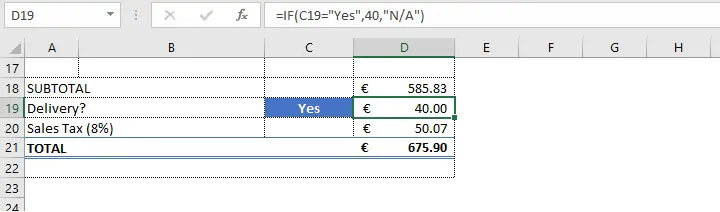If the value_if_false argument is omitted, a FALSE value is returned if cell C19 says “No”.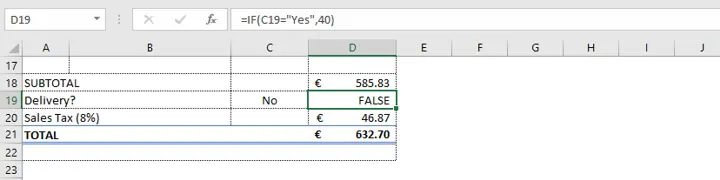## Return a mathematical calculation

The IF function can also be set to return a mathematical calculation instead of a specific value. For example, if a delivery cost of 10% of the subtotal is to be charged instead of 40 euros, the value_if_true can be expressed as a formula. The input in cell D19 would be:

````=IF(C19="Yes”,D18*0.10,“N/A”)`
```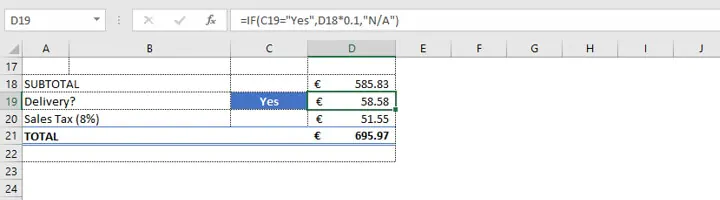## Nested Excel IF statements

We can use nested IF functions to build a single formula that accounts for different scenarios. A nested statement is one where one function is used as the argument of another function. Expanding on our previous example, we could have two cells being evaluated in order to determine the outcome of cell D19:

1. If cell C19 is equal to the word “Yes” then cell D19 should calculate and return a value that is 10% of the value in D18.
2. If cell C19 is not equal to the word “Yes” then cell D19 should return a value of “N/A”.
3. If cell D18 (the subtotal) is greater than 500 euros, the delivery fee is automatically waived. Cell D19 should return the words “Fee Waived”.

The order in which Excel evaluates and applies these statements is important since the first TRUE condition will override each subsequent condition. Therefore, nested IF functions should be listed in order of priority.

In this case, if the subtotal is greater than 500 euros, then waiving the delivery fee will override the 10% calculation.

Stated separately, the Excel statements would be:

``IF(C19=“Yes”,D18*0.10, “N/A”)``
``IF(D18>500, “Fee Waived”)``

Represented as a decision tree, the above logic would look like this: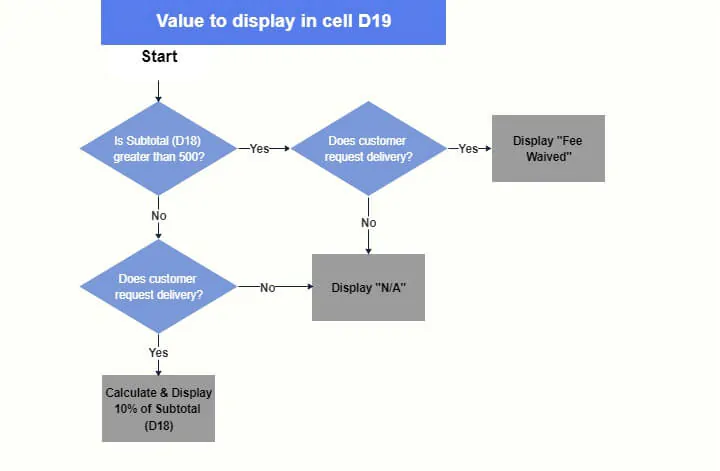The correct way to nest both IF statements is as follows:

``=IF(D18>500, “Fee Waived”, IF(C19= “Yes”, D18*0.10, “N/A”))``

The statement with the lower priority becomes the value_if_false of the previous statement. The three possible outcomes are shown below.

Scenario 1 - Subtotal is greater than 500 euros and customer requests delivery.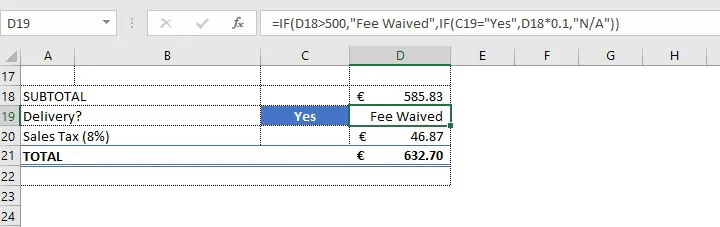Scenario 2 - Subtotal is not greater than 500 euros and customer requests delivery.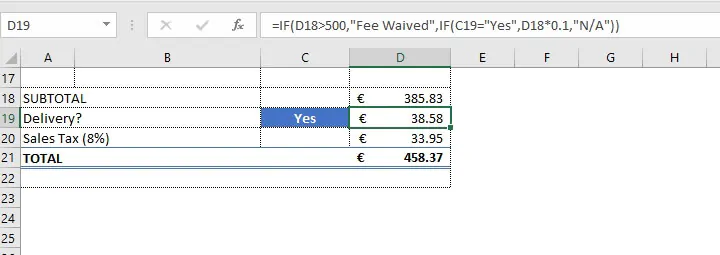Scenario 3 - Subtotal is not greater than 500 euros and customer does not request delivery.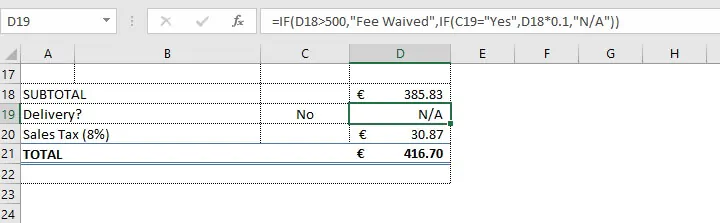Of course, there is also a fourth scenario. The customer’s subtotal may have been greater than 500 euros, but no delivery was requested. Since the value in the Subtotal cell is considered the overriding consideration, then “Fee Waived” will be displayed in cell D19, whether or not C19 is equal to “Yes”. The outcome would be the same as that in Scenario one.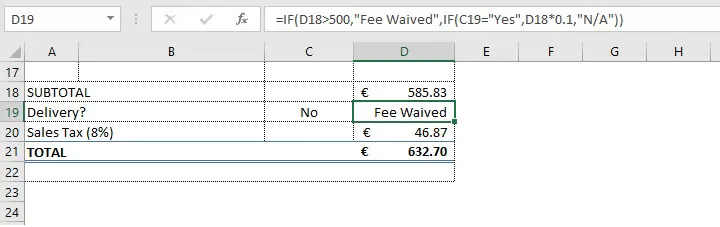## IF combined with OR, AND

The IF function also enables customized responses when nested with the OR and AND functions rather than the default TRUE or FALSE return values.

## Example 2

In the example below, students get a “Pass” or “Fail” result depending on their letter grade. An A, B or C grade is considered a Pass, and a D or F is a Fail.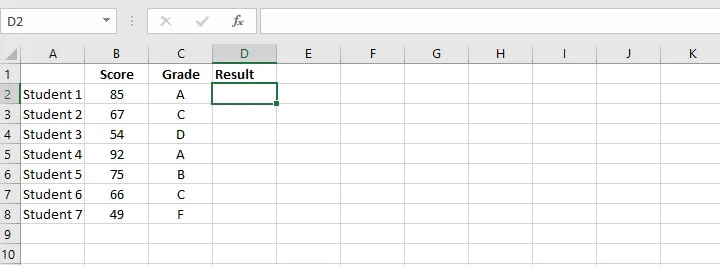An OR statement would seek to determine if at least one of the following conditions is true:

• C2 = A
• C2 = B
• C2 = C

This simple formula would be written:

``=OR(C2=“A”,C2=“B”,C2=“C”)``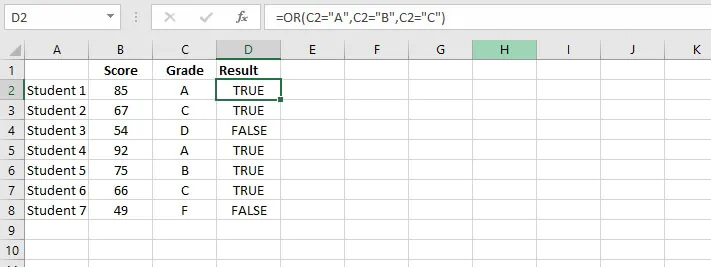If at least one condition is true, then C2 passes the logical test, and TRUE will be returned in cell D2. If not, a value of FALSE will be returned. Instead of the generic TRUE/FALSE result, placing an IF statement before the OR statement above means that the OR formula itself becomes the logical_test of the IF function.

``=IF(OR(C2=“A”,C2=“B”,C2=“C”), “Pass”, “Fail”)``

In the above nested IF/OR formula, “Pass” is the value_if_true and “Fail” is the value_if_false.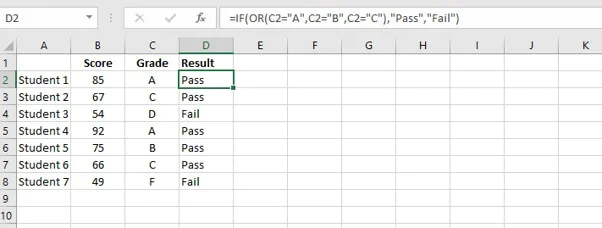## Example 3

The format for nesting the IF/AND functions is similar. In the example below, students who receive 85 or higher on both their quiz and final exam are considered outstanding.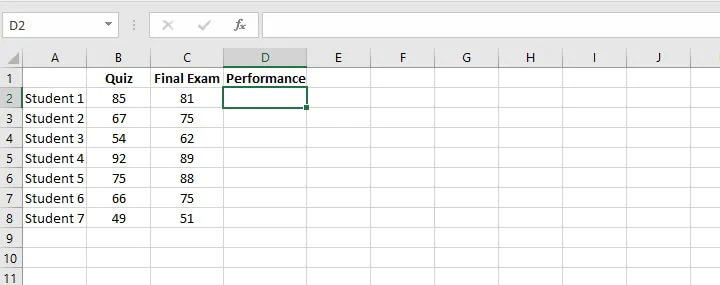Since both criteria must be met, the AND function would be nested with the IF function in order to customize the output in column D.

The text “Outstanding” is returned if both conditions are met. If one or both conditions fail, the value_if_false can be set to return an empty string, represented by empty double quotes.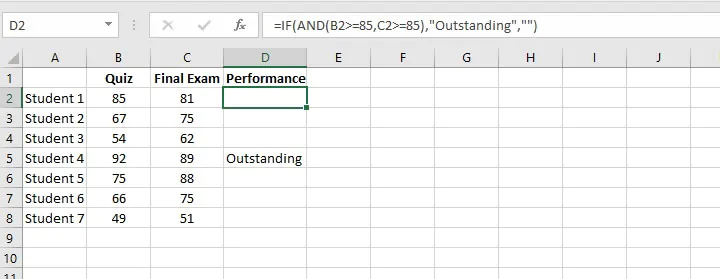If you intend to use Excel extensively, the Excel IF function is definitely one you’ll want to get comfortable with. In fact, there’s a whole family of IF functions just waiting to make your life a whole lot easier.

To learn more essential Excel functions try our Microsoft Excel - Basic and Advanced course today. Or start with some formula basics with our free Excel in an Hour course.

Start learning formulas, functions, and time-saving hacks today with this free course!#### Join the Excel conversation on Slack

Ask a question or join the conversation for all things Excel on our Slack channel.Claudia Buckley

Claudia is a project manager and business skills instructor at GoSkills. In her spare time, she reads mystery novels and does genealogy research.